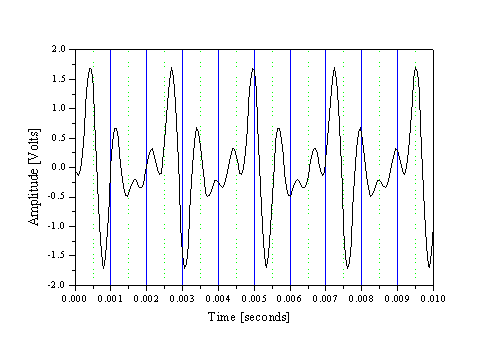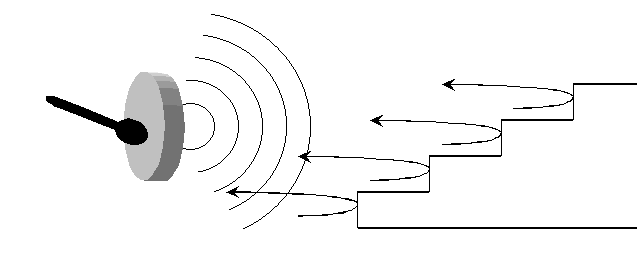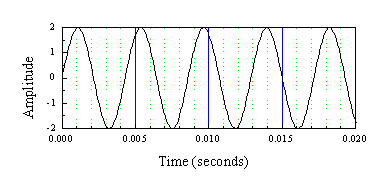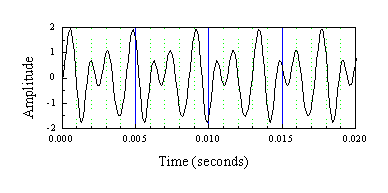2.1 Pitch, Frequency, Period, Loudness, Timbre

To begin our discussion, we will consider the attributes or characteristics of any kind of wave. Each of these characteristics can be associated with something that we hear in a sound wave.

The most noticeable aspect of a wave is that it repeats in time. Whether it is a vibrating string on a violin or waves breaking at the shore, something is repeating. Each repetition is called an oscillation:

An oscillation is one segment of a repetitive motion.

Pitch, Frequency, Period

Musical notes or tones have a pitch. The pitch of a particular note is often given as a number. For example, the note "A" in the middle of a piano is designated A=440. Now, the question is 440 what? This number is how many oscillations occur in 1 second.

If you pluck a violin string tuned to Middle A, the string will vibrate or oscillate back and forth and will have a certain pitch. The technical term for pitch is frequency and the frequency referred to here is how many times in one second the string oscillates back and forth:

Pitch of Middle A corresponds to a frequency of 440 oscillations in 1 second.

Physicists do not like to keep write expressions out like this all of the time, so a shorthand has been developed. We can write,

A 440 = 440 oscillations per second = 440 oscillations/second = 440/sec = 440 Hz.

Each way of writing this gets progressively more compact. Since frequency always refers to some number of oscillations, we do not have to keep writing "oscillations". Also, "per second" is more easily written as /second, and second is abbreviated as sec. What may be more unfamiliar is the designation that "/sec" = "Hz". Hz is an abbreviation of the unit Hertz, named after the physicists Heinrich Hertz. 1 Hertz corresponds to 1 oscillation/second.

Once we understand the meaning of a pitch or frequency of 440 Hz, we can ask a related question: how long does 1 oscillation of the vibrating string take? If the string oscillates 440 times in 1 second, then each oscillation will take (1/440) seconds. Another way to look at this is the following: if each oscillation takes (1/440) seconds then 440 oscillations will take 1 second. This is the same as saying that there are 440 oscillations/second.

So, the time it takes for 1 oscillation is called the period, and the period is related to the frequency by:

Period = 1/frequency

For the example of a pitch of 440 Hz, the period will be:

1/(440/sec) = (1/440) sec = 0.00227 sec

= 2.27 millisecond

= 2.27 msec.

Again, we have used some shorthand notation. If the period is rather small, we don't want to keep writing lots of zeros after the decimal point, so we use scientific notation, instead. 10-3 seconds corresponds to 1 millisecond and 1 millisecond is abbreviated as 1 msec. This is especially convenient for sound waves, as the periods of sound waves are generally around 1 to 100 msec.

EXAMPLES

1. Consider the Earth going around the sun. Is this motion repetitive? What would an oscillation correspond to? What is the period and frequency of the motion?

2. What is the period and frequency of the wave on the following graph?Repetitive sounds can be formed in different ways. The most common, of course, is from a musical instrument. But, now, consider the situation where you are standing in front of a set of bleachers and you strike a bass drum:The individual echoes of the drum off each step are delayed from one another. So, to the drummer the echoes form a repetitive sound which then is heard as a pitch. Anyone who has played in a marching band can attest to this!

Amplitude - Loudness

Besides the pitch of a musical note, perhaps the most noticeable feature in how loud the note is. The loudness of a sound wave is determined from its amplitude. While loudness is only associated with sound waves, all types of waves have an amplitude. Waves on a calm ocean may be less than 1 foot high. Good surfing waves might be 10 feet or more in amplitude. During a storm the amplitude might increase to 40 or 50 feet.

Many things can influence the amplitude.

1. What is producing the sound?
2. How far are you from the source of the sound? The farther away the smaller the amplitude.
3. Intervening material. Sound does not travel through walls as well as air.
4. Depends on what is detecting the wave sound. Ear vs. microphone.

Shape of the repetitive motion.

Although a wave repeats in time, its motion during on oscillation can be simple or highly complex. For example, the following two graphs each show repetitive motion and the period and frequency of the motion is the same in both cases.In fact, their amplitudes are also the same. Thus, if these two waves represented sound waves, the pitch and loudness would be the same in both cases. But would they sound exactly the same? The answer is No, because there is one more attribute to sound waves that you are familiar with, and that is tone quality. This is what makes different instruments sound different. A violin and a trumpet can play the same pitch with the same loudness, but we can easily tell them apart, because they have a different tone quality. In fact, the same instrument can create different tone qualities. If you pluck a guitar in different ways, you can get quite different tones. Try it! The technical musical term for this is timbre. For general waves, we refer to the shape of the wave or sometimes the waveform.

In the first lab, you will experiment with all three characteristics of sound waves: frequency, dependence of loudness on distance from the sound source, and timbre.